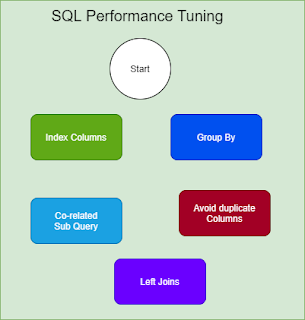### The Quick and Easy Way to Analyze Numpy ArraysThe quickest and easiest way to analyze NumPy arrays is by using the numpy.array() method. This method allows you to quickly and easily analyze the values contained in a numpy array. This method can also be used to find the sum, mean, standard deviation, max, min, and other useful analysis of the value contained within a numpy array. Sum You can find the sum of Numpy arrays using the np.sum() function.  For example:  import numpy as np  a = np.array([1,2,3,4,5])  b = np.array([6,7,8,9,10])  result = np.sum([a,b])  print(result)  # Output will be 55 Mean You can find the mean of a Numpy array using the np.mean() function. This function takes in an array as an argument and returns the mean of all the values in the array.  For example, the mean of a Numpy array of [1,2,3,4,5] would be  result = np.mean([1,2,3,4,5])  print(result)  #Output: 3.0 Standard Deviation To find the standard deviation of a Numpy array, you can use the NumPy std() function. This function takes in an array as a par

# Five top SQL Query Performance Tuning Tips

SQL query runs faster when you write it in a specific method. You can say it as tuning. There are five tuning tips:

## List of Performance Tuning Tips

1. use index columns,
2. use group by,
3. avoid duplicate column in SELECT & Where,
4. use Left Joins
5. use a co-related subquery.Five top SQL Query Performance Tuning Tips

## SQL Performance Tuning Tip: 01

Use indexes in the where clause of SQL. Let me elaborate more on that. Be sure the columns that you are using in the WHERE clause should be already part of the Index columns of that database Table.

An example SQL Query:

SELECT
FROM emp_sal_nonppi
WHERE dob <= 2017-08-01;

## SQL Performance Tuning Tip: 02

Use GROUP BY. Some people use a DISTINCT clause to eliminate duplicates. You can achieve this by GROUP BY.

An example SQL Query:

SELECT E.empno, E.lastname
FROM emp E,emp_projact EP
WHERE E.empno = EP.empno
GROUP BY E.empno, E.lastname;

## SQL Performance Tuning Tip: 03

Avoid using duplicates in the Query. Some people use the same column name in both WHERE & SELECT statements, which is not a good practice since it takes more CPU time.

An example SQL Query:

SELECT dept-no, dept-name
FROM dept
WHERE (admrdept = 'E01' OR deptno = 'D01')
AND deptname = 'MAIN BRANCH';

## SQL Performance Tuning Tip: 04

There are occasions you need data from more than one Table. You can achieve this by joining two or more tables.

Left Join is much faster than Right join. See the Sets concept. There are many kinds of joins. Out of these LEFT and RIGHT outers popular in SQL. The SQL parser converts all types of joins to the left outer joins. So to avoid extra CPU time prefer to use LEFT Joins, which are faster.

An example SQL Query:

SELECT column_names FROM table1
LEFT JOIN table2
ON table1.common_column = table2.common_column;Tuning tips

## SQL Performance Tuning Tip: 05

This idea is from SQL gurus that always use index columns in correlated-Subquery. In any SQL query, you can use normal sub-queries and Correlated-subqueries.

If a sub-query is referring to the columns of the Outer query, then you can say that query as correlated-subquery. Otherwise, it is a normal sub-query. The columns referenced in correlated-subquery should be index columns.

An example SQL Query:

SELECT last_name, first_name
FROM employee e1
WHERE NOT EXISTS (SELECT ph.last_name
FROM payment_history ph
WHERE ph.employee_id = e1.employee_id
AND ph.payment_type = 'award';